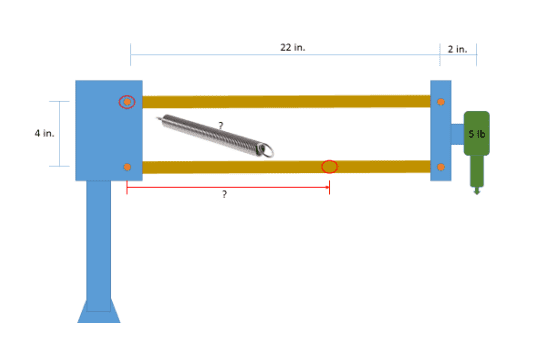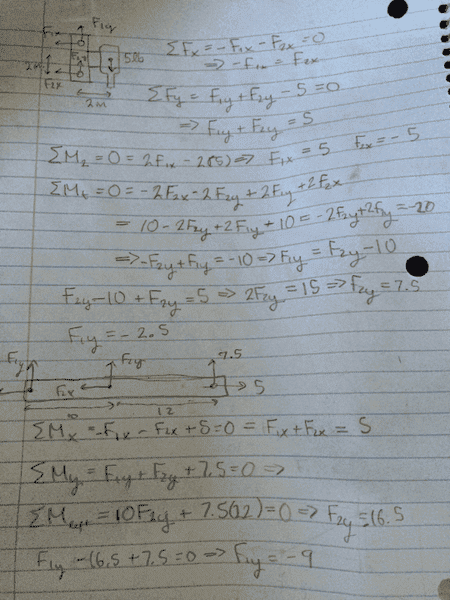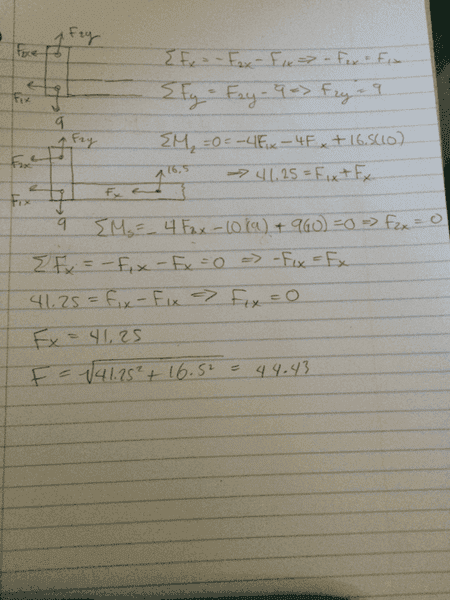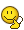# Finding force needed for spring to balance load

• Triathlete

## Homework Statement

I am given a tool that needs to be balanced in the horizontal position with a spring of my design. I need to know the force that the spring will need to exert in order to do this. I am also choosing the mount point, which will be at 10 in.## Homework Equations

Fsy = veritical component of spring force
Fsx = horizontal component of spring force
Ft = force of 5 lb tool

## The Attempt at a Solution

ΣFy = 0 = Fsy - 5 ⇒ Fsy = 5 lb
ΣMtool = 0 = (2)(Fsx) + (24 - 10)(Fsy) ⇒ Fsx = - 35 lb

I know this can't be right because that force is to large. I'm probably making some really fundamental errors, so if anyone could help that would be fantastic.
Thanks!

Your diagram shows four rigid bodies. Which one do your equations relate to?
Whichever it is, there should be forces from the joints with other bodies.

Does it matter where you position the spring? E.g. are you supposed to minimise the needed stiffness of the spring?

The moment was taken on the lower bar about the midpoint of the tool. It doesn't matter where I position the spring, I simply chose 10 at random just to see if I was finding the force correctly.

The moment was taken on the lower bar about the midpoint of the tool. It doesn't matter where I position the spring, I simply chose 10 at random just to see if I was finding the force correctly.
There is an upper bar, a lower bar, the tool on the right and a stand on the left. Four bodies, three of which can potentially move. Each exerts a force on two of the others, in addition to the forces from the spring. You do not have nearly enough variables in your equations.

I went section by section to try and figure it out, and ended up with the resultant force of the spring being about 44 lb. Not sure where I'm going wrong.I went section by section to try and figure it out, and ended up with the resultant force of the spring being about 44 lb. Not sure where I'm going wrong.
View attachment 99617 View attachment 99618
I don't understand your diagrams. I do not see the spring represented.

Sorry, I labelled the forces but didn't actually show the spring. The middle force in the second FBD and the far right force in the last FBD are both the x and y components of the forces on the spring. The top left forces on the third FBD is the first mount point of the spring.
Even if the forces I got were correct, I'm not sure how to answer the original problem statement. I assumed solving for the force at the second mount point would answer it, because the first mount point should have the same force but in the opposite direction. However, that is not turning out to be true with my answers(which are most likely incorrect), so I am questioning if that assumption is even valid, and what I actually need to find.

Sorry, I labelled the forces but didn't actually show the spring. The middle force in the second FBD and the far right force in the last FBD are both the x and y components of the forces on the spring. The top left forces on the third FBD is the first mount point of the spring.
Even if the forces I got were correct, I'm not sure how to answer the original problem statement. I assumed solving for the force at the second mount point would answer it, because the first mount point should have the same force but in the opposite direction. However, that is not turning out to be true with my answers(which are most likely incorrect), so I am questioning if that assumption is even valid, and what I actually need to find.
Looks like you changed all the dimensions, and it's not clear what some of them refer to in the thumbnail sketches, so I cannot check that aspect of your equations.
Where does the second moments equation in the first page (Mt?) come from? I cannot figure out what axis it relates to.
In principle, there is no way to tell how the vertical load is distributed between the upper and lower supports. E.g. you can imagine that at the lower support the horizontal rod merely rests against the upright, all the vertical load being taken by the upper support.

Mt is the moment about the center point of the 5lb tool on the right end.
As far as dimensions, they are the same, although I suppose with the way I broke the diagrams into sections it may not look like they correlate very well. The mount point distance of 10 inches in my diagrams isn't in the original picture either, it's shown as a question mark.
Any advice as to the direction that I should take to solve this? I've been working on this specific portion of the problem(which is part 4 of 11 parts) for a few days now and I can't seem to get it right. Is there any other method I should use(perhaps relating to spring design equations)? It seems like it should be more simplistic because I'm supposed to have Excel do multiple iterations to find the force with the changing mount point distance.
Thanks for all of your help!

Mt is the moment about the center point of the 5lb tool on the right end.
As far as dimensions, they are the same, although I suppose with the way I broke the diagrams into sections it may not look like they correlate very well. The mount point distance of 10 inches in my diagrams isn't in the original picture either, it's shown as a question mark.
Any advice as to the direction that I should take to solve this? I've been working on this specific portion of the problem(which is part 4 of 11 parts) for a few days now and I can't seem to get it right. Is there any other method I should use(perhaps relating to spring design equations)? It seems like it should be more simplistic because I'm supposed to have Excel do multiple iterations to find the force with the changing mount point distance.
Thanks for all of your help!
In the original diagram you have an overall height of 4" and width of 24". In the first hand drawn diagram there is a width of 2m. The height might be 2m or 4m, it is not clear. I tried to deduce that from the algebra but it looks like 2 in one place and 2+2 in another.

In your Mt equation, you have a wrong sign on one of the vertical forces. F1y and F2y have the same moment about the tool. That is why you managed to deduce the values of those two forces when, as a matter of principle you cannot. You can only find their total value.
Another principle worth knowing is that you can only get 3 independent statics equations for one rigid body. Usually it is two linear and one for moments, but other combinations are possible. So your fourth equation (Mt) cannot tell you anything more than you already had in the first three.

Moving on to your second FBD, I take this to be the lower horizontal bar.
Looks like you have flipped left and right, compared to the original diagram, which is most confusing. Assuming so, your two forces 5 and 7.5 are wrong. Think in what directions those two forces act on the bar.

The 2m is just the distance to the midpoint, half of the 4m.
My main question is am I going about finding the answer to the question in the correct manner or is there some other method I should be using?
Thanks

The 2m is just the distance to the midpoint, half of the 4m.
Half of what 4m? The original diagram has 4 inches, not 4 metres. And the horizontal distance to the tool is 24 inches, not 2 metres.
My main question is am I going about finding the answer to the question in the correct manner
The method will work, but you are making lots of errors in executing it.
An easier path may be to think about work. Suppose the spring is not quite tense enough, so the horizontal bars drop through a small angle theta. What work balance equation can you write?